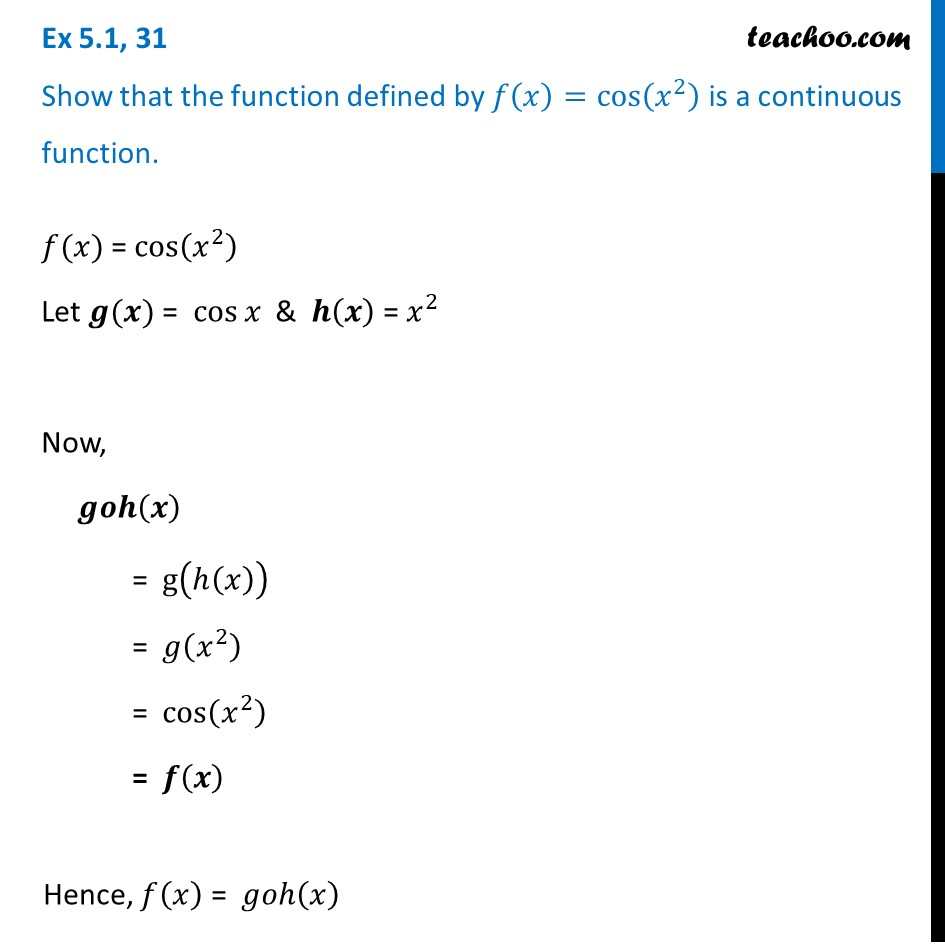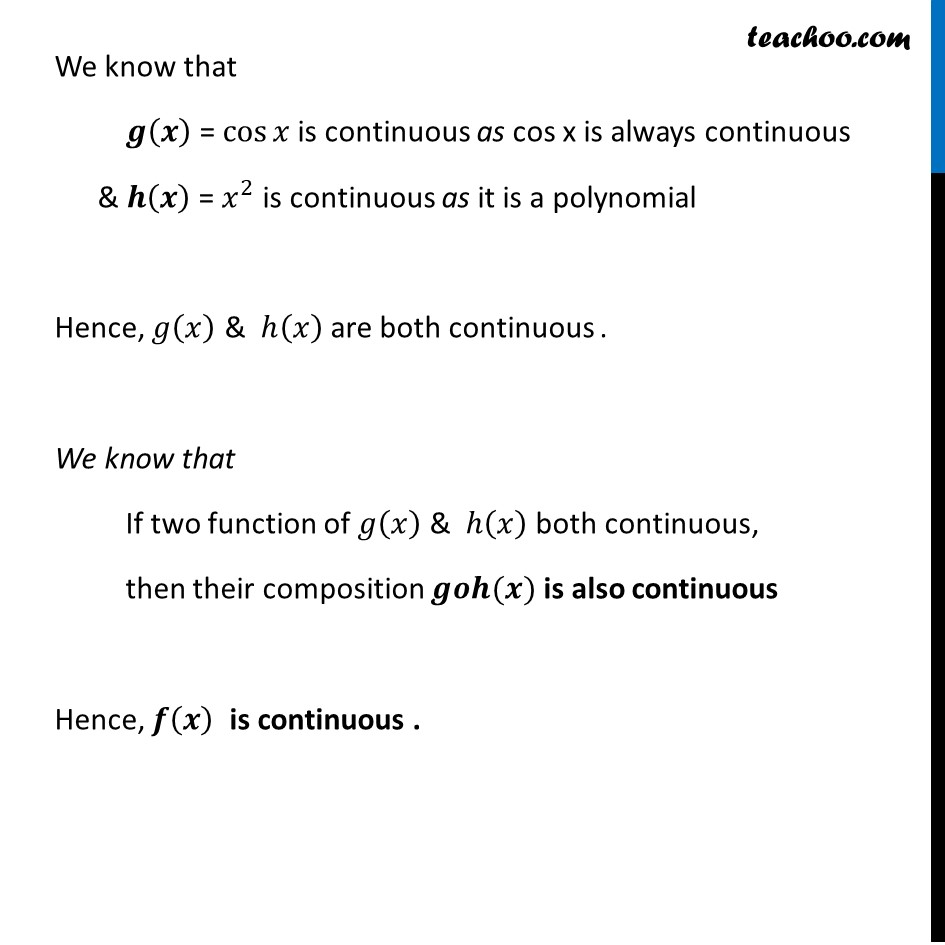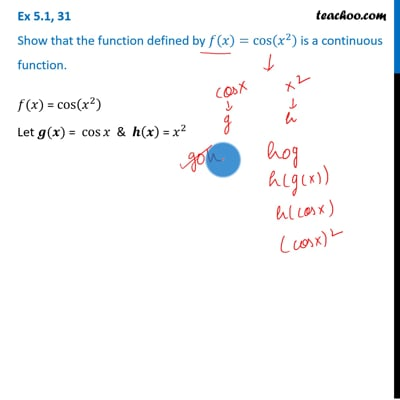Ex 5.1

Chapter 5 Class 12 Continuity and Differentiability
Serial order wiseThis video is only available for Teachoo black users

Introducing your new favourite teacher - Teachoo Black, at only ₹83 per month

### Transcript

Ex 5.1, 31 Show that the function defined by 𝑓(𝑥)=cos⁡(𝑥^2 ) is a continuous function.𝑓(𝑥) = cos⁡(𝑥^2 ) Let 𝒈(𝒙) = cos⁡𝑥 & 𝒉(𝒙) = 𝑥^2 Now, 𝒈𝒐𝒉(𝒙) = g(ℎ(𝑥)) = 𝑔(𝑥^2 ) = cos⁡(𝑥^2 ) = 𝒇(𝒙) Hence, 𝑓(𝑥) = 𝑔𝑜ℎ(𝑥) We know that 𝒈(𝒙) = cos⁡𝑥 is continuous as cos x is always continuous & 𝒉(𝒙) = 𝑥^2 is continuous as it is a polynomial Hence, 𝑔(𝑥) & ℎ(𝑥) are both continuous . We know that If two function of 𝑔(𝑥) & ℎ(𝑥) both continuous, then their composition 𝒈𝒐𝒉(𝒙) is also continuous Hence, 𝒇(𝒙) is continuous .